NEET  >  Short & Long Answer Questions: Kinetic Theory

# Short & Long Answer Questions: Kinetic Theory - Physics Class 11 - NEET

Q.1 Mention the different ways of increasing the number of molecular collisions per unit time in a gas.

Answer: The number of collisions per unit time can be increased by

(i) increasing the temperature of the gas,

(ii) increasing the number of molecules, and

(iii) decreasing the volume of the gas.
Q.2 What is an ideal gas? Give its main characteristics.

Answer: An ideal gas is one which obeys the gas laws at all values of temperature and pressure. Its main characteristics are:-

(i) The size of the molecules is negligibly small.

(ii) There is no force of attraction or repulsion among its molecules.
Q.3 Why do the gases at low temperature and high pressure show large deviations from ideal behaviour?

Answer: At low temperature and high pressure, the inter-molecular attractions become appreciable. Moreover, the volume occupied by the gas molecules cannot be neglected in comparison to the volume of the gas. Hence the real gases show large deviations from ideal gas behaviour.
Q.4 On reducing the volume of a gas at constant temperature, the pressure of the gas increases. Explain it on the basis of kinetic theory.

Answer: On reducing the volume, the number of molecules per unit volume increases. Hence a large number of molecules collide with the walls of the vessel per second and a larger momentum is transferred to the wall per second. This increases the pressure of the gas.

Q.5 When an automobile travels for a long distance, the air pressure in the tyres increases slightly. Why?

Answer: Due to the friction between the tyres and the road, the tyres get heated. The temperature of air inside the tyres increases. Consequently, the air pressure in the tyres increases slightly.
Q.6 When a gas is heated, its temperature increases. Explain it on the basis of kinetic theory of gases.

Answer: When a gas is heated, the root mean square velocity of its molecules increases. As rms∝√T, so the temperature of the gas increases.
Q.7 In the upper part of the atmosphere the kinetic temperature of air is of the order of 1000 K, event then one feels severe cold there. Why?

Answer: As we go up in the atmosphere, the number of air molecules per unit volume decreases. The quantity of heat per unit volume or the heat density is low. But the translational kinetic energy per molecule is quite large. As the kinetic temperature is the measure of translational kinetic energy, so the kinetic temperature is quite high in the upper atmosphere but one feels severe cold there due to low heat density.
Q.8 In the kinetic theory of gases, why do we not take into account the changes in gravitational potential energy of the molecule?

Answer: The changes in gravitational potential energy are negligibly small as compared to the mean kinetic energy of molecules.
Q.9 What type of motion is associated with the molecules of a gas?

Answer: Brownian motion. In this motion any particular molecule will follow a zig-zag path due to the collisions with the other molecules or with the walls of the container.
Q.10 Although the root-mean-square speed of gas molecules is of the order of the speed of sound in that gas, yet on opening a bottle of ammonia in one corner of a room its smell takes time in reaching the other corner. Why?

Answer: The molecules of ammonia have random motion. They frequently collide with one another. Consequently, their net speed in any particular direction is low. So gas takes several seconds to go from one corner to the other corner of the room.
Q.11  On which factors does the average kinetic energy of gas molecules depend: Nature of the gas, temperature, volume?

Answer: The average K.E.of a gas molecule depends only on the absolute temperature of the gas and is directly proportional to it.
Q.12 What do you mean by the r. m. s. speed of the molecules of a gas? Is r. m. s. speed same as the average speed?

Answer: The r. m. s. speed of the molecules of a gas is defined as the square root of the mean of the squared velocities of the molecules of a gas. No, r. m. s. speed is different from the average speed. For example,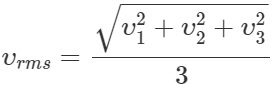and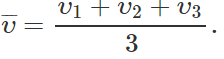Q.13 The ratio of vapour densities of two gases at the same temperature is 8:9. Compare the r. m. s. velocities of their molecules.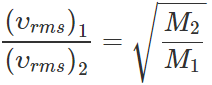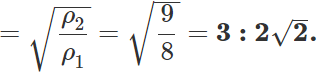Q.14 What is the average velocity of the molecules of an ideal gas?

Answer: The average velocity of the molecules of an ideal gas is zero, because the molecules possess all sorts of velocities in all possible directions so their vector sum and hence average is zero.
Q.15 Given a sample of 1 cm3 of hydrogen and 1 cmof oxygen both at S.T.P. Which sample has a larger number of molecules?

Answer: Both the samples contain the same number of molecules in accordance with Avogadro's law.
Q.16 At what temperature does all molecular motion cease? Explain.
Or
Molecular motion ceases at zero kelvin. Explain.

Answer: All molecular motion ceases at absolute zero or at 0 K. According to the kinetic interpretation of temperature,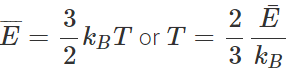or absolute temperature ∝ average K.E. of molecules ∴ The temperature = 0 K, average K.E. = 0. Thus at 0 K, the velocity of molecules becomes zero.
Q.17 Why temperature less than absolute zero is not possible?

Answer: According to the kinetic interpretation of temperature, Absolute temperature ∝ average K.E. of molecules As the heat is removed, the temperature falls and velocity of molecules decreases. At absolute zero, the molecular motion eases i.e., the kinetic energy becomes zero. As kinetic energy cannot be negative, so no further decrease in kinetic energy is possible. Hence temperature cannot be decreased below 0K.
Q.18 For an ideal gas, the internal energy can only be translational kinetic energy. Explain.

Answer: In an ideal gas, the molecules can be considered as point masses with no intermolecular forces between them. So there can neither be internal potential energy nor the internal energy due to rotation or vibration. The molecules can have only translational kinetic energy.
Q.19 At a given temperature, equal masses of monatomic and diatomic gases are supplied equal quantities of heat. Which of the two gases will suffer a larger temperature rise?

Answer: The temperature of the monatomic gas will rise by a large value. In case of the monatomic gas, the heat supplied is used entirely to increase the translational K.E. of the molecules. In case of the diatomic gas, the heat supplied is used to increase the translational, rotational and sometimes even the vibrational kinetic energy of the molecules. It is only the translational K.E. which increases the temperature.
Q.20 Deduce the dimensional formula for R used in the ideal gas equation PV=nRT.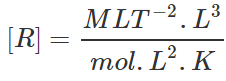=[ML2T−2K−1mol−1].
Q.21 A box contains equal number of molecules of hydrogen and oxygen. If there is a fine hole in the box, which gas will leak rapidly? Why?

Answer: As υrms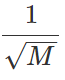Hence hydrogen gas will leak more rapidly because of its smaller molecular mass.
Q.22 The mass of a molecule of krypton is 2.25 times the mass of a hydrogen molecule. A mixture of equal masses of these gases is enclosed in a vessel. Calculate at any temperature the ratio of the root mean square velocities of krypton and hydrogen gases.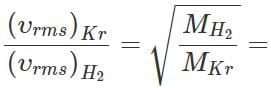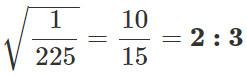Q.23 A sample of an ideal gas occupies a volume V at a pressure P and absolute temperature T. The mass of each molecule is m. If kB is the Boltzmann's constant, then write the expression for the density of the gas.

Answer: According to kinetic theory of gases,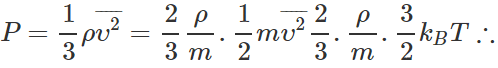Density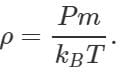Q.24 The volume of vessel A is twice the volume of another vessel B, and both of them are filled with the same gas. If the gas in A is at twice the temperature and twice the pressure in comparison to the gas in B, what is the ratio of the gas molecules in A and B?

PV=nRT ∴  nB=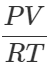and nA=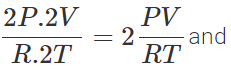nA:nB=2:1
Q.25 Two gases, each at temperature T, volume V and pressure P are mixed such that the temperature and volume of the mixture are T and V respectively. What would be the pressure of the mixture? Justify your answer on the basis of kinetic theory.

Answer: From kinetic theory,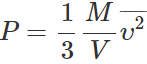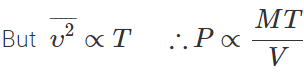As both T and V remain unchanged but mass M is doubled, so the pressure of mixture gets doubled i.e., it is equal to 2P.
Q.26 The total translational kinetic energy of the molecules of a gas having volume V and pressure P is 500 J. What will be the total translational kinetic energy of the molecules of the same gas occupying the same volume V but exerting a pressure 2P?

Answer: If E is the translational K.E. per unit volume, then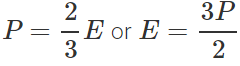Total translational K.E. of volume V is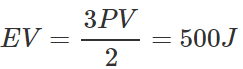When pressure becomes 2P, total translational K.E. =2×500=1000J

Q.27 Write the equation of state for 16 g of O2.

Answer: Number of moles in 32g of O2=1 ∴Number of moles in 16 g of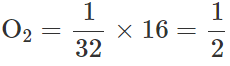As PV=nRT and n=1/2,,so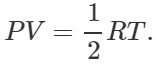Q.28 When a gas filled in a closed vessel is heated through 1C, its pressure increases by 0.4. What is the initial temperature of the gas?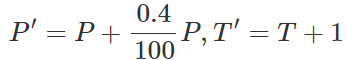By Gay Lussac's law,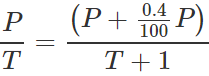On solving, T=250K.
Q.29 A gas in a closed vessel is at the pressure P0 . If the masses of all the molecules be made half and their speeds be made double, then find the resultant pressure.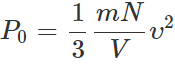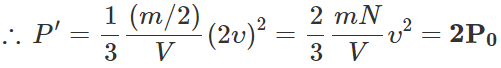Q.30  What is evaporation?

Answer: All the molecules do not have the same velocity. The molecules which possess large velocities are able to overcome the molecular attraction and escape the liquid surface, which is known as evaporation.
Q.31 Why does evaporation cause cooling?

Answer: The evaporation of a liquid from its surface occurs because some molecules acquire velocities sufficient enough to escape from the attractive force at the surface. Because escaping molecules have higher kinetic energy, hence the average kinetic energy of the molecules left behind decreases. As average kinetic energy is directly related with temperature, hence evaporation causes cooling.

Q.32 Cooking gas containers are kept in a lorry moving with uniform speed. What will be the effect on temperature of the gas molecules?

Answer: As the lorry is moving with a uniform speed, there will be no change in the translational motion or K.E. of the gas molecules. Hence the temperature of the gas will remain same.
Q.33 Should the specific heat of monatomic gas be less than, equal to or greater than that of a diatomic gas at room temperature? Justify your answer.

Answer: The specific heat of a gas at constant volume is equal to f/2 R. For monatomic gases, f=3, so CV=3/2 R. For diatomic gases,    f=5,so CV=5/2 R. Hence the specific heat for monatomic gas is less than that for a diatomic gas.
Q.34 What is Debye temperature?

Answer: The temperature above which the molar specific heat of a solid becomes equal to 6 cal mol−1K−1is called Debye temperature.
Q.35 Equal masses of helium and oxygen gases are given equal quantities of heat. Which gas will undergo a greater temperature rise?

Answer: Helium is a monatomic gas, while oxygen is diatomic. Therefore the heat given to helium will be totally used up in increasing the translational kinetic energy of its molecules; whereas the heat given to oxygen will be used up in increasing the translational kinetic energy of its molecules and also in increasing the kinetic energy of rotation and vibration. Hence, there will be a greater rise in the temperature of helium.
Q.36 A gas is filled in a cylinder fitted with piston at a constant temperature. Explain on the basis of kinetic theory: (i) The pressure of the gas increases by raising the temperature. (ii) On pulling the piston out, the pressure of the gas decreases.

Answer: (i) On raising the temperature, the average speed of the gas molecules increases. As a result, the molecule collide more frequently with the walls of the vessel and also greater momentum is transferred to the wall in each collision. Both of these factors increase the pressure of the gas.

(ii) When the piston is pulled out, the volume of the gas increases. The molecules have to traverse a large distance to collide with the walls of the cylinder. So a lesser number of molecules collides with the walls per second. Moreover, the collisions now occur on a larger area of the walls. Both of these factors decrease the pressure of the gas.
Q.37 There are N molecules of a gas in a container. If the number of molecules is increased to 2N, what will be (i) pressure of the gas, (ii) total energy of the gas and (ii) r. m. s. speed of the gas?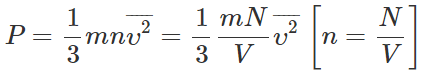(i) As P∝N,so the pressure of the gas is doubled when the number of molecules is increased from N to 2N.

(ii) Average K.E. per molecule,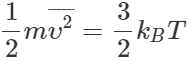Total energy of N molecules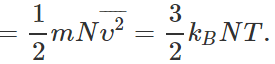When the number of molecules is increased from N to 2N, total energy of the gas is doubled, though the average K.E. per molecule remains same. (iii) The r. m. s. speed remains same because it depends only upon temperature.
Q.38 Although the velocity of air molecules is nearly 0.5 kms−1, yet the smell of scent spreads at a much slower rate. Why?

Answer: The air molecules travel along a zig-zag path due to frequent collisions. As a result, their displacement per unit time is very small. Hence the smell of scent spreads very slowly.
Q.39 When do the real gases obey more correctly the gas equation: PV=nRT?

Answer: An ideal gas is one whose molecules have zero volume and no mutual force between them. At low pressure, the volume of a gas is large and so the volume occupied by the molecules is negligible in comparison to the volume of the gas. At high temperature, the molecules have large velocities and so the inter-molecular force has no influence on their motion. Hence at low pressure and high temperature, the behaviour of real gases approach the ideal gas behaviour.
Q.40 Explain qualitatively how the extent of Brownian motion is affected by the (a) size of the Brownian particle, (b) density of the medium, (c) temperature of the medium, (d) viscosity of the medium?
Answer: The effect of the various factors on the Brownian motion is as follows:

 Factors Effects (a) Decrease in the size of the Brownian particle. Increase of Brownian motion. (b) Decrease in the density of the medium. Increase of Brownian motion. (c) Increase in temperature of the medium. Increase of Brownian motion. (d) Increase in viscosity of the medium. Decrease of Brownian motion.

Q.41 For Brownian motion of particles of suspensions in liquids, what should be the typical size of suspended particles? Why should not the size of the particles be too small (say of atomic dimensions 10−10m ) or too large (say of the order of 1m)?

Answer: The typical size of the suspended particles should be 10−6m. If the size of the suspended particles is too small (≈10−10m), the net momentum imparted to the suspended particle due to bombardment of neighbouring fluid particles would be zero and hence no Brownian motion is possible. If the size of the suspended particle is too large (≈1m), the particle will not move due to its large inertia, even when an unbalanced force is acting on it.
Q.42 What is the simplest evidence in nature that you can think of to suggest that atoms are not point particles?

Answer: Brownian motion is the simplest evidence in nature to suggest that atoms are not point particles but have finite size. The particles suspended in a fluid move in the direction of the unbalanced force due to the unequal bombardment caused by the atoms or molecules of the fluid in which they are suspended. This shows that the atoms have finite size.
Q.43 What is meant by molar specific heat of a gas? The molar specific heat of hydrogen H2 is about 5/2 R in the temperature range of about 250 K to 750 K. At lower temperatures, molar specific heat of hydrogen decreases to the value typical of monatomic gases: 3/2 R. At higher temperatures, it tends to the value 7/2 R. What do you think is happening?

Answer: The molar specific heat of a gas is defined as the amount of heat required to raise the temperature of 1 mole of a gas through 1C. The molar specific heat of a gas at constant volume is given by CV=f/2 R,, where f is degrees of freedom of the gas. In the range of about 250 to 750 K,, a diatomic gas such as H2 gas possesses 5 degrees of freedom (3 corresponding to translational and 2 corresponding to rotational modes of motion Of the gas). Hence CV=5/2 R (between 250 to 750 K) But at lower temperatures, the rotational motion is not excited and hydrogen gas molecule possesses only 3 degrees of freedom corresponding to translational motion. Hence at lower temperatures,  CV=3/2 R At higher temperatures, hydrogen gas   molecule has 2 additional degrees of freedom corresponding to vibrational mode of the motion, so that total degrees of freedom become f=5+2=7. Hence at higher temperatures, CV=7/2 R.
Q.44 (a) When a molecule (or an elastic ball) hits a (massive) wall, it rebounds with the same speed. When a ball hits a massive bat held firmly, the same thing happens. However, when the bat is moving towards the ball/the ball rebounds with a different speed. Does the ball move faster or slower? (b) When gas in a cylinder is compressed by pushing in a piston, its temperature rises. Guess at an explanation of this in terms of kinetic theory using (a) above. (c) What happens when a compressed gas pushes a piston out and expands? What would you observe? (d) Sachin Tendulkar uses a heavy cricket bat while playing. Does it help him in any way?

Answer: (a) Let the speed of the ball be u relative to the wicket behind the bat. If the bat is moving towards the ball with a speed V relative to the wicket, then the relative speed of the ball to bat is V +u towards the bat. When the ball rebounds (after hitting the massive bat) its speed, relative to bat,V+(V+u)=2V+u, moving away from the wicket. So the ball speeds up after the collision with the bat. For a molecule this would imply an increase in temperature.

(b) When a gas in cylinder is compressed by pushing in a piston, the speed of the molecules or their kinetic energy increases. This increases the temperature of the gas.

(c) When a compressed gas pushes a piston out, the speed of the molecules or their kinetic energy decreases. This decreases the temperature of the gas.

(d) When the ball is hit with a heavy bat, the rebound speed of the ball further increases. It helps to score better.

The document Short & Long Answer Questions: Kinetic Theory | Physics Class 11 - NEET is a part of the NEET Course Physics Class 11.
All you need of NEET at this link: NEET

## Physics Class 11

116 videos|451 docs|188 tests

## FAQs on Short & Long Answer Questions: Kinetic Theory - Physics Class 11 - NEET

 1. What is kinetic theory?Ans. Kinetic theory is a scientific model that explains the behavior of particles in matter. It states that all matter is composed of tiny particles (atoms or molecules) that are in constant motion and have kinetic energy.
 2. How does kinetic theory explain the properties of gases?Ans. According to kinetic theory, gas particles are in constant random motion and collide with each other and the walls of the container. This motion explains the properties of gases, such as their ability to fill the entire container, their high compressibility, and their low density.
 3. What is the relationship between temperature and the kinetic energy of particles?Ans. According to kinetic theory, the temperature of a substance is directly proportional to the average kinetic energy of its particles. As the temperature increases, the particles move faster and have higher kinetic energy.
 4. Can kinetic theory be applied to solids and liquids as well?Ans. Yes, kinetic theory can be applied to solids and liquids as well. Although the particles in solids and liquids have less freedom of motion compared to gases, they still possess kinetic energy and undergo random motion. However, the arrangement and forces between particles in solids and liquids are more ordered and stronger than in gases.
 5. How does kinetic theory explain the concept of pressure in gases?Ans. Kinetic theory explains pressure in gases by considering the collisions between gas particles and the walls of the container. When gas particles collide with the container walls, they exert a force, and the cumulative effect of these collisions leads to the macroscopic property of pressure. The more frequent and energetic the collisions, the higher the pressure.

## Physics Class 11

116 videos|451 docs|188 testsExplore Courses for NEET exam### How to Prepare for NEET

Read our guide to prepare for NEET which is created by Toppers & the best Teachers
Signup to see your scores go up within 7 days! Learn & Practice with 1000+ FREE Notes, Videos & Tests.
10M+ students study on EduRev
Track your progress, build streaks, highlight & save important lessons and more!
Related Searches

,

,

,

,

,

,

,

,

,

,

,

,

,

,

,

,

,

,

,

,

,

;